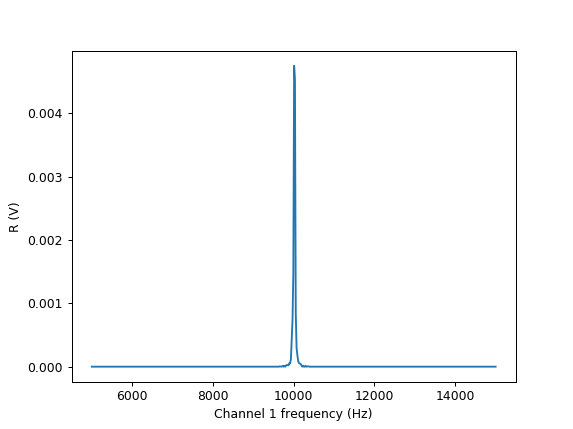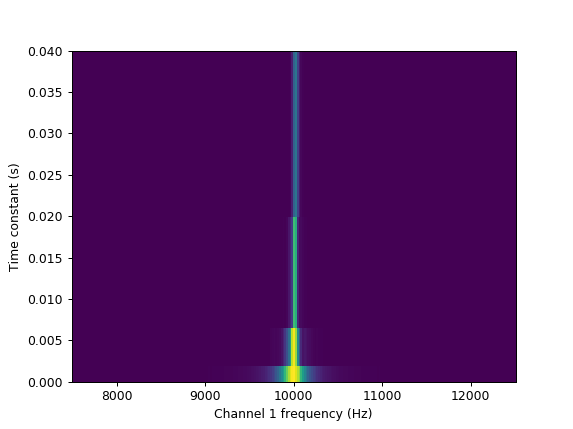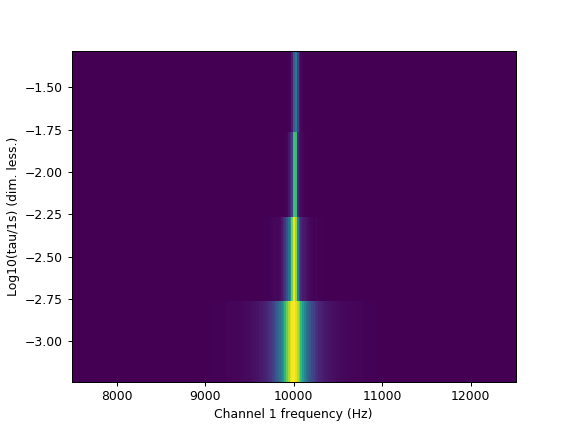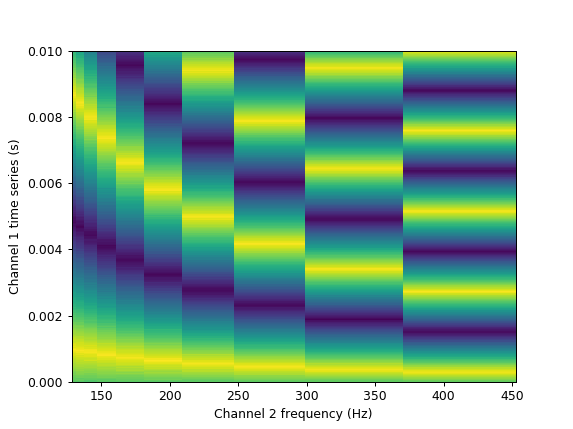This page was generated from docs/examples/DataSet/Real_instruments/Example Measurements with Real Instruments.ipynb. Interactive online version:.

# Example Measurements with Real Instruments

It’s good to have 100% test coverage, but it’s better to have actual instruments performing measurements.

Here we run some example measurements on the QCoDeS test setup rack using the new dataset’s Measurement object. No drivers were modified to make this notebook run; the new dataset is indeed compatible with the existing drivers.

:

%matplotlib notebook
from time import sleep
import numpy as np
import qcodes as qc
from qcodes.dataset.measurements import Measurement
from qcodes.dataset.plotting import plot_by_id

# Drivers
from qcodes.instrument_drivers.Keysight.KeysightAgilent_33XXX import WaveformGenerator_33XXX
from qcodes.instrument_drivers.stanford_research.SR830 import SR830
from qcodes.instrument_drivers.Keysight.Infiniium import Infiniium

:

# initialisation

sr830 = SR830('SR830', 'GPIB0::8::INSTR')
key = WaveformGenerator_33XXX('Keysight', 'TCPIP0::K-33522B-00256::inst0::INSTR')
mso = Infiniium('MSO', 'TCPIP0::AGILENT-32C1770::inst0::INSTR')

station = qc.Station(key, mso, sr830)

qc.new_experiment(name='EMwRI', sample_name='no_sample')

\\tsclient\sourcecodes\qcodes\qcodes\instrument\parameter.py:209: UserWarning: Wrapping get method, original get method will not be directly accessible. It is recommended to define get_raw in your subclass instead.
warnings.warn('Wrapping get method, original get method will not '

Connected to: Stanford_Research_Systems SR830 (serial:s/n48280, firmware:ver1.07) in 0.91s
Connected to: Agilent Technologies 33522B (serial:MY57800256, firmware:3.05-1.19-2.00-52-00) in 0.15s
Connected to: Agilent Technologies MSO8104A (serial:MY45001438, firmware:05.71.0000) in 0.08s

:

EMwRI#no_sample#14@./experiments.db
-----------------------------------


## Measurement 1: Frequency sweeps

We output a sine from the function generator to the lock-in. While sweeping the sine frequency, we record the demodulated R. We also play around a bit and vary the time constant.

:

###
# Instrument preparation

# Keysight
key.ch1.function_type('SIN')
key.ch1.amplitude_unit('VPP')
key.ch1.amplitude(0.01)
key.ch1.offset(0)
key.ch1.frequency(1e3)  # this will be varied below

# SR830
sr830.ch1_display('R')
sr830.time_constant(0.01)
sr830.sensitivity(0.1)
sr830.frequency(10e3)

:

# set-up and tear-down actions
def setup():
key.ch1.output('ON')

def teardown():
key.ch1.output('OFF')

# simple frequency sweep

meas = Measurement()
meas.register_parameter(key.ch1.frequency)
meas.register_parameter(sr830.R, setpoints=(key.ch1.frequency,))

tau = sr830.time_constant()

with meas.run() as datasaver:
for freq in np.linspace(5e3, 15e3, 500):
key.ch1.frequency(freq)
sleep(tau)  # The SR830 does not wait by itself for the integration time to pass
R = sr830.R()
(sr830.R, R))

run_id = datasaver.run_id

plot = plot_by_id(run_id)

Starting experimental run with id: 35:

# Sweeping frequency and varying time constant

meas = Measurement()
meas.register_parameter(key.ch1.frequency)
meas.register_parameter(sr830.time_constant)
meas.register_parameter(sr830.R, setpoints=(key.ch1.frequency,
sr830.time_constant))

with meas.run() as datasaver:

run_id = datasaver.run_id

taus = [0.001, 0.003, 0.01, 0.03]
freqs = np.linspace(7.5e3, 12.5e3, 250)

for tau in taus:
sr830.time_constant(tau)
for freq in freqs:
key.ch1.frequency(freq)
sleep(tau)
R = sr830.R()
(key.ch1.frequency, freq),
(sr830.R, R))

plot = plot_by_id(run_id)

Starting experimental run with id: 36Looking at the above plot, we might rather want to record the log10 of the time constant. This is easy!

:

# Sweeping frequency and varying time constant, recording the logarithm

meas = Measurement()
meas.register_parameter(key.ch1.frequency)
meas.register_custom_parameter(name='log_tau', label='Log10(tau/1s)', unit='dim. less.')
meas.register_parameter(sr830.R, setpoints=(key.ch1.frequency,
'log_tau'))

with meas.run() as datasaver:
run_id = datasaver.run_id

taus = [0.001, 0.003, 0.01, 0.03]
freqs = np.linspace(7.5e3, 12.5e3, 250)

for tau in taus:
sr830.time_constant(tau)
for freq in freqs:
key.ch1.frequency(freq)
sleep(tau)
R = sr830.R()
(key.ch1.frequency, freq),
(sr830.R, R))

plot = plot_by_id(run_id)

Starting experimental run with id: 37## Measurement 2: Triangle Traces

We record triangle pulses on an oscilloscope. We continuously emit triangle pulses, trigger, read one of them, and then sweep up the frequency.

:

# Prepare the scope
def scope_setup():
mso.ch1.input('DC50')

for i in range(4):
# turn of all channels
mso.channels[i].display(False)

# switch channel 1 back on
mso.ch1.display(True)
mso.timebase_range(10e-3)
mso.timebase_position(0.5*mso.timebase_range())
mso.ch1.range(1.1)

nPoints = 10000
mso.acquire_points(nPoints)
mso.acquire_sample_rate(nPoints/mso.timebase_range())

mso.acquire_average(False)
mso.trigger_enabled(True)
mso.trigger_sweep('triggered')
mso.trigger_edge_source('CHANnel1')
mso.trigger_edge_slope('positive')
mso.ch1.trigger_level(0.1)
mso.trigger_sweep('single')

mso.ch1.trace.prepare_curvedata()

mso.write('run')

def keysight_setup():
# Prepare the Keysight
key.ch2.function_type('TRI')
key.ch2.amplitude(0.5)
key.ch2.frequency(1.3e2)
key.ch2.burst_state('OFF')
key.ch2.output('ON')

def keysight_teardown():
key.ch2.output('OFF')

:

meas = Measurement()
meas.register_parameter(key.ch2.frequency)
meas.register_parameter(mso.ch1.trace, setpoints=(key.ch2.frequency,))
# the setpoints of the arrayparameter are registered automatically

mso.ch1.trace.prepare_curvedata()
with meas.run() as datasaver:
for freqfactor in np.linspace(1, 1.25, 10):
freq = key.ch2.frequency()*freqfactor
key.ch2.frequency(freq)
trace = mso.ch1.trace.get()

Starting experimental run with id: 40[ ]: